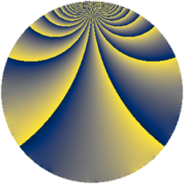# Properties

 Label 441.2.gLevel $441$ Weight $2$ Character orbit 441.g Rep. character $\chi_{441}(67,\cdot)$ Character field $\Q(\zeta_{3})$ Dimension $72$ Newform subspaces $8$ Sturm bound $112$ Trace bound $5$

# Related objects

## Defining parameters

 Level: $$N$$ $$=$$ $$441 = 3^{2} \cdot 7^{2}$$ Weight: $$k$$ $$=$$ $$2$$ Character orbit: $$[\chi]$$ $$=$$ 441.g (of order $$3$$ and degree $$2$$) Character conductor: $$\operatorname{cond}(\chi)$$ $$=$$ $$63$$ Character field: $$\Q(\zeta_{3})$$ Newform subspaces: $$8$$ Sturm bound: $$112$$ Trace bound: $$5$$ Distinguishing $$T_p$$: $$2$$, $$5$$

## Dimensions

The following table gives the dimensions of various subspaces of $$M_{2}(441, [\chi])$$.

Total New Old
Modular forms 128 88 40
Cusp forms 96 72 24
Eisenstein series 32 16 16

## Trace form

 $$72 q - q^{2} + q^{3} - 33 q^{4} + 10 q^{5} + 2 q^{6} - 12 q^{8} - q^{9} + O(q^{10})$$ $$72 q - q^{2} + q^{3} - 33 q^{4} + 10 q^{5} + 2 q^{6} - 12 q^{8} - q^{9} + 6 q^{10} - 6 q^{11} - 19 q^{12} + 3 q^{13} + 10 q^{15} - 27 q^{16} - 9 q^{17} + 19 q^{18} - 4 q^{20} - 8 q^{23} - 12 q^{24} + 42 q^{25} - 16 q^{26} + 7 q^{27} - 18 q^{29} + 72 q^{30} + 3 q^{31} + 41 q^{32} + 16 q^{33} - 70 q^{36} + 3 q^{37} + 38 q^{38} - 28 q^{39} - 12 q^{40} - 10 q^{41} - 11 q^{44} + 4 q^{45} - 12 q^{46} - 27 q^{47} + 5 q^{48} - 45 q^{50} - 14 q^{51} - 30 q^{52} + 16 q^{53} + 62 q^{54} + 6 q^{55} - 15 q^{57} - 18 q^{58} - 30 q^{59} - 16 q^{60} + 12 q^{62} + 12 q^{64} + 30 q^{65} + 41 q^{66} + 6 q^{67} + 60 q^{68} - 6 q^{69} + 6 q^{71} - 51 q^{72} - 12 q^{73} - 82 q^{74} - 43 q^{75} - 6 q^{76} + 69 q^{78} + 18 q^{79} - 19 q^{80} - q^{81} - 18 q^{83} + 3 q^{85} - 50 q^{86} - 29 q^{87} + 18 q^{88} - 41 q^{89} - 25 q^{90} - 52 q^{92} + 58 q^{93} + 3 q^{94} - 17 q^{95} - 14 q^{96} + 3 q^{97} - 34 q^{99} + O(q^{100})$$

## Decomposition of $$S_{2}^{\mathrm{new}}(441, [\chi])$$ into newform subspaces

Label Dim $A$ Field CM Traces $q$-expansion
$a_{2}$ $a_{3}$ $a_{5}$ $a_{7}$
441.2.g.a $2$ $3.521$ $$\Q(\sqrt{-3})$$ None $$-1$$ $$3$$ $$2$$ $$0$$ $$q+(-1+\zeta_{6})q^{2}+(2-\zeta_{6})q^{3}+\zeta_{6}q^{4}+\cdots$$
441.2.g.b $6$ $3.521$ $$\Q(\zeta_{18})$$ None $$-3$$ $$0$$ $$-6$$ $$0$$ $$q+(-1+\zeta_{18}+\zeta_{18}^{5})q^{2}+(\zeta_{18}^{2}+\zeta_{18}^{4}+\cdots)q^{3}+\cdots$$
441.2.g.c $6$ $3.521$ $$\Q(\zeta_{18})$$ None $$-3$$ $$0$$ $$6$$ $$0$$ $$q+(-1+\zeta_{18}+\zeta_{18}^{5})q^{2}+(-\zeta_{18}^{2}+\cdots)q^{3}+\cdots$$
441.2.g.d $6$ $3.521$ 6.0.309123.1 None $$1$$ $$-2$$ $$10$$ $$0$$ $$q+(-\beta _{1}-\beta _{2}-\beta _{3}+\beta _{5})q^{2}+(-1+\cdots)q^{3}+\cdots$$
441.2.g.e $6$ $3.521$ 6.0.309123.1 None $$1$$ $$2$$ $$-10$$ $$0$$ $$q+(-\beta _{1}-\beta _{2}-\beta _{3}+\beta _{5})q^{2}+(1-\beta _{1}+\cdots)q^{3}+\cdots$$
441.2.g.f $10$ $3.521$ 10.0.$$\cdots$$.1 None $$2$$ $$-2$$ $$8$$ $$0$$ $$q+\beta _{1}q^{2}+(-\beta _{2}+\beta _{7})q^{3}+(-1-\beta _{2}+\cdots)q^{4}+\cdots$$
441.2.g.g $12$ $3.521$ $$\mathbb{Q}[x]/(x^{12} - \cdots)$$ None $$-2$$ $$0$$ $$0$$ $$0$$ $$q+(-\beta _{2}+\beta _{5})q^{2}+(-\beta _{8}-\beta _{10})q^{3}+\cdots$$
441.2.g.h $24$ $3.521$ None $$4$$ $$0$$ $$0$$ $$0$$

## Decomposition of $$S_{2}^{\mathrm{old}}(441, [\chi])$$ into lower level spaces

$$S_{2}^{\mathrm{old}}(441, [\chi]) \cong$$ $$S_{2}^{\mathrm{new}}(63, [\chi])$$$$^{\oplus 2}$$